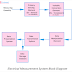Hey, in this article we are going to see the basic electrical measurement system block diagram. From this block diagram, you can easily understand, how an electrical measurement system works. First of all, let's know what is electrical measurement. Basically, electrical measurement is a system or method, or device that is used to measure different types of electrical quantity such as voltage, current, frequency, resistance, and physical quantities such as temperature, pressure, force, etc. In an electrical measurement system, the electrical quantities can be measured directly but when measuring quantities, they should be converted into electrical quantities using transducers then it should be measured.

First of all, let's see the generalized block diagram of electrical measurement system.

Here, the main parts or blocks of an electrical measurement system are explained below.

### 1. Measuring Quantity

It may be a physical quantity or electrical quantity is to be measured. The physical quantity required more conversion and special sensing process whereas electrical quantity does not require more conversion and sensing elements.

### 2. Primary Sensing Element

It is the ground-level component that actually communicates or is used to measure the element is to be measured. It may be a sensor or transducer. In most of the cases, a transducer is used. The transducer is a device that can convert the physical quantity into an electrical quantity or electrical signal. So the measuring element first comes to contact with the primary sensing element.

### 3. Variable Conversion Element

It is involved in the signal conversion process. For example, the transducer produces analog signals but the circuit needs digital signals. In this case, the analog signal is converted into a digital signal through the conversion element. Not only does the analog to digital or digital to analog process happen in the conversion element, but the signal is converted for different purposes and different forms such as suitable for controlling, manipulating, recording, presenting, etc.

### 4. Data or Variable Manipulation Element

Here, signal manipulation happens. Here, signal amplification, compression, modulation, etc happen. That means it does not change any variable or data from the signal provided by the previous element. It just increases the magnitude of the signal or compresses to reduce transmission hazard, etc. This is also known as the data conditioning element or signal conditioning element.

### 5. Data Transmission System

The data transmission system consists of a pathway for data transmission and data transmission elements. For a large and remote measurement system, the data transmission system helps a lot. In some measurement systems, this section is not used. This section is also known as the intermediate stage o the system.

### 6. Data Presentation System

Here, the data or information is presented in front of the user. Users can see all the data here in an arranged way. So it can be helpful for measuring, analyzing, etc. Different types of output devices, display devices are used for data presentation systems. In the data presentation system, the output devices may work with analog signals or digital signals. In most of the cases, the output devices required digital signals only.

### 7. Data Storage Element

Sometimes data is to be stored or record to analyze information over a long time. For this purpose memory element or data, the storage element can be used. The data storage element store the data from the manipulation element and supplies it to the display or presentation element when required.

So, this was the basic concept of an electrical measurement system. Nowadays there are so many advanced systems, devices are available in the market that measures with very high accuracy. Also, they have very complex circuitry. There are two types of measurement - high precision measurement and less precise measurement. High precise measurements are mostly done in laboratories such as measurement of the speed of light, the charge of the electrons, etc whereas the low precision measurements are mostly performed on daily basis in industries, and productions, etc.

Understand Electrical Measurement System with Block DiagramReviewed by Author on 11:03 am Rating: 5